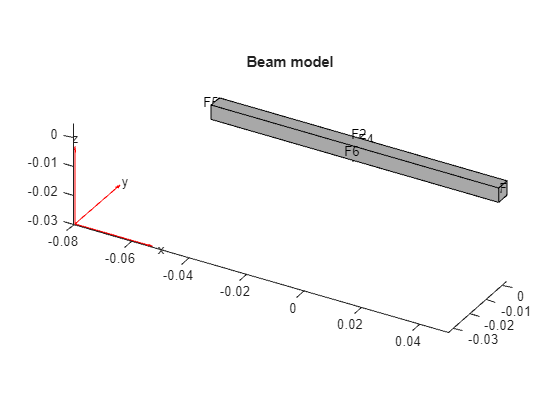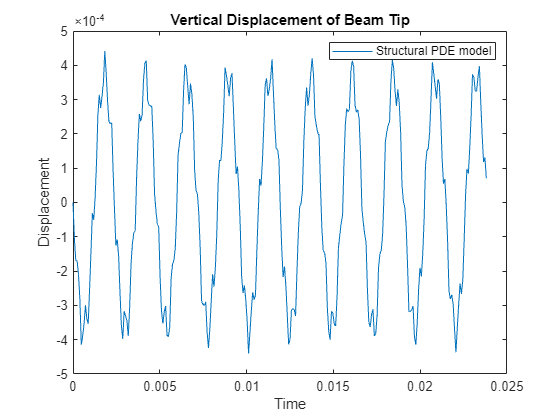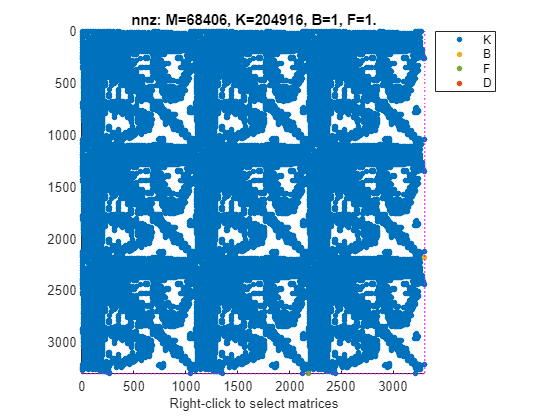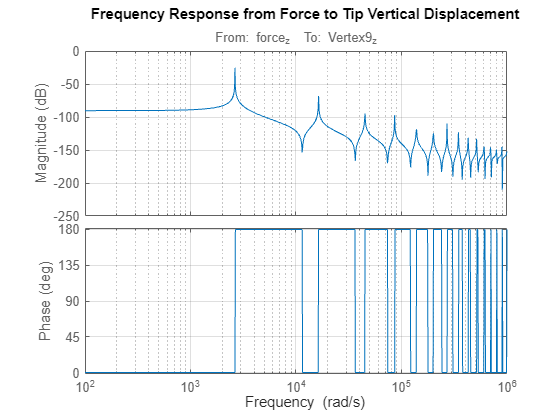# Linear Analysis of Cantilever Beam

This example shows how to obtain a structural model of a beam and calculate the time and frequency response when it is subjected to an impulse force. For this example, consider a steel cantilever beam subject to a point load at the tip. Building the structural model requires Partial Differential Equation Toolbox™.

The steel beam is deformed by applying an external load of 100 N at the tip of the beam and then released. This example does not use any additional loading, so the displacement of the tip oscillates with an amplitude equal to the initial displacement from the applied force. This example follows the three-step workflow:

1. Construct a structural model of the beam.

2. Linearize the structural model to obtain a sparse linear model of the beam.

3. Analyze the time and frequency response of the linearized model.### Structural Model of Beam

Using Partial Differential Equation Toolbox, build a structural model and compute the impulse response. For an example of modeling a cantilever beam, see Dynamics of Damped Cantilever Beam (Partial Differential Equation Toolbox).

First construct the beam and specify the Young's modulus, Poisson's ratio, and mass density of steel. Specify the tip of the beam using the `addVertex` function.

```gm = multicuboid(0.1,0.005,0.005); E = 210E9; nu = 0.3; rho = 7800; TipVertex = addVertex(gm,'Coordinates',[0.05,0,0.005]);```

Use `createpde` (Partial Differential Equation Toolbox) to construct the structural model and generate the mesh using the `generateMesh` (Partial Differential Equation Toolbox) command.

```sModel = createpde('structural','transient-solid'); sModel.Geometry = gm; msh = generateMesh(sModel);```

Visualize the beam geometry using `pdegplot` (Partial Differential Equation Toolbox).

```figure pdegplot(sModel,'FaceLabels','on'); title('Beam model')```Assign structural properties for the steel beam with the `structuralProperties` (Partial Differential Equation Toolbox) command and fix one end using `structuralBC` (Partial Differential Equation Toolbox).

```structuralProperties(sModel,'YoungsModulus',E,'PoissonsRatio',nu,'MassDensity',rho); structuralBC(sModel,'Face',5,'Constraint','fixed');```

You can calculate the vibration modes of the beam by solving the modal analysis model in a specified frequency range using `solve` (Partial Differential Equation Toolbox). For this beam, the first vibration mode is at 2639 rad/s as confirmed by the Bode plot in the Linear Analysis section of this example.

```firstNF = 2639; Tfundamental = 2*pi/firstNF;```

To model an impulse (knock) on the tip of the beam, apply force for 2% of the fundamental period of oscillation (impulse) using `structuralBoundaryLoad` (Partial Differential Equation Toolbox). Specify the label `force` to use this force as a linearization input.

```Te = 0.02*Tfundamental; structuralBoundaryLoad(sModel,'Vertex',TipVertex,... 'Force',[0;0;-100],'EndTime',Te,'Label','force');```

Set initial conditions for the beam model using `structuralIC` (Partial Differential Equation Toolbox).

`structuralIC(sModel,'Velocity',[0;0;0]);`

Compute the impulse response by solving the structural beam model.

```ncycles = 10; tsim = linspace(0,ncycles*Tfundamental,30*ncycles); R1 = solve(sModel,tsim);```

Visualize the oscillations at the tip of the beam.

```figure plot(tsim,R1.Displacement.uz(TipVertex,:)) title('Vertical Displacement of Beam Tip') legend('Structural PDE model') xlabel('Time') ylabel('Displacement')```### Structural Model Linearization

For this beam model, you want to obtain a linear model (transfer function) from the force applied at the tip to the z-displacement of the tip.

To do so, first specify the inputs and outputs of the linearized model in terms of the structural model. Here, the input is the force specified with `structuralBoundaryLoad` (Partial Differential Equation Toolbox) and the output is the z degree of freedom of the tip vertex.

```linearizeInput(sModel,'force'); linearizeOutput(sModel,'Vertex',TipVertex,'Component','z');```

Then, use the `linearize` (Partial Differential Equation Toolbox) command to extract the `mechss` model.

`sys = linearize(sModel)`
```Sparse continuous-time second-order model with 1 outputs, 3 inputs, and 3303 degrees of freedom. Model Properties Use "spy" and "showStateInfo" to inspect model structure. Type "help mechssOptions" for available solver options for this model. ```

The linearized model has three inputs corresponding to the x, y, and z components of the force applied to the tip vertex.

`sys.InputName`
```ans = 3×1 cell {'force_x'} {'force_y'} {'force_z'} ```

In the linearized model, select the z component of the force.

`sys = sys(:,3)`
```Sparse continuous-time second-order model with 1 outputs, 1 inputs, and 3303 degrees of freedom. Model Properties Use "spy" and "showStateInfo" to inspect model structure. Type "help mechssOptions" for available solver options for this model. ```

The resultant model is defined by the following set of equations:

`$\mathit{M}\stackrel{¨}{\mathit{q}}+\mathit{Kq}=\mathit{B}×\mathit{force}$`

`$\mathit{y}=\mathit{Fq}$`

Use `spy` to visualize the sparsity of the `mechss` model `sys`.

```figure spy(sys)```### Linear Analysis

Choose `'tfbdf3'` as the differential algebraic equation (DAE) solver.

`sys.SolverOptions.DAESolver = 'trbdf3';`

Use `bode` to compute the frequency response of the linearized model `sys`.

```w = logspace(2,6,1000); figure bode(sys,w) grid title('Frequency Response from Force to Tip Vertical Displacement')```The Bode plot shows that the first vibration mode is at approximately `firstNF `= 2639 rad/s.

Next use `lsim` to simulate the impulse response and compare with the results from the PDE model. To limit the error due to linear interpolation of the force between samples, use a step size of `Te/5`. Recall that the force is applied at the beam tip for the short time interval [0 `Te`].

```h = Te/5; t = 0:h:ncycles*Tfundamental; u = zeros(size(t)); u(t<=Te) = -100; y = lsim(sys,u,t); figure plot(t,y,tsim,R1.Displacement.uz(TipVertex,:)) title({'Comparison of Sparse LSIM Response';... 'with PDE Model Simulation'}) legend('Linearized model','Structural PDE model') xlabel('Time') ylabel('Displacement')```The linear response from `lsim` closely matches the transient simulation of the structural model obtained in the first step.